##### The Japanese Bonsai specialist
Direct order Contact Help / Services Newsletter# Rotating working table green t

› Bonsai tools › Bonsai tools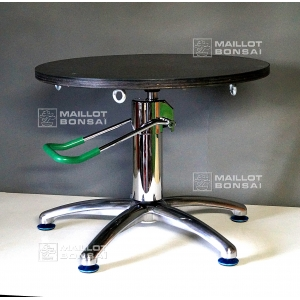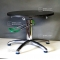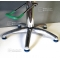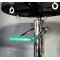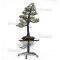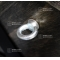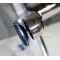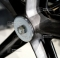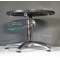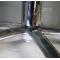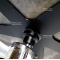ref. : 10178

385,00

This item is temporarily unavailableNotify availability

###### Description

Diameter of the table: 580 mm, thickness: 20 mm. Made in Italy, this beautifully finished plywood and metal table is for bonsai enthusiasts of every level. The foot pedal, operated by a hydraulic jack, allows the height to be adjusted between 470 and 820 mm. It can bear a 200 kilo weight on low position and 40 kilos on high position.. Directional feet offer better stability while you work on the smallest to the biggest bonsai. The rotating table can be blocked by pressing the handle. Five hooks allow the bonsai pot to be fixed to the table. Sold without bonsai nor bungee cords. This portable table weighs 16 kilos and can be carried by its handle.

#table 7.5 #bonsai 6.6 #rotating 3.8 #position 2.8 #working 2.7 #handle 2.6 #green 2.5 #tools 2.5 #kilos 2.5 #this 2.4

Formule
(( ROUND((CHAR_LENGTH(b.article_nom)-CHAR_LENGTH(REPLACE(b.article_nom, 'table', '')))/LENGTH('table')) + ROUND((CHAR_LENGTH(b.article_description)-CHAR_LENGTH(REPLACE(b.article_description, 'table', '')))/LENGTH('table')) ) * 7.5) + (( ROUND((CHAR_LENGTH(b.article_nom)-CHAR_LENGTH(REPLACE(b.article_nom, 'bonsai', '')))/LENGTH('bonsai')) + ROUND((CHAR_LENGTH(b.article_description)-CHAR_LENGTH(REPLACE(b.article_description, 'bonsai', '')))/LENGTH('bonsai')) ) * 4.6) + (( ROUND((CHAR_LENGTH(b.article_nom)-CHAR_LENGTH(REPLACE(b.article_nom, 'rotating', '')))/LENGTH('rotating')) + ROUND((CHAR_LENGTH(b.article_description)-CHAR_LENGTH(REPLACE(b.article_description, 'rotating', '')))/LENGTH('rotating')) ) * 3.8) + (( ROUND((CHAR_LENGTH(b.article_nom)-CHAR_LENGTH(REPLACE(b.article_nom, 'position', '')))/LENGTH('position')) + ROUND((CHAR_LENGTH(b.article_description)-CHAR_LENGTH(REPLACE(b.article_description, 'position', '')))/LENGTH('position')) ) * 2.8) + (( ROUND((CHAR_LENGTH(b.article_nom)-CHAR_LENGTH(REPLACE(b.article_nom, 'working', '')))/LENGTH('working')) + ROUND((CHAR_LENGTH(b.article_description)-CHAR_LENGTH(REPLACE(b.article_description, 'working', '')))/LENGTH('working')) ) * 2.7) + (( ROUND((CHAR_LENGTH(b.article_nom)-CHAR_LENGTH(REPLACE(b.article_nom, 'handle', '')))/LENGTH('handle')) + ROUND((CHAR_LENGTH(b.article_description)-CHAR_LENGTH(REPLACE(b.article_description, 'handle', '')))/LENGTH('handle')) ) * 2.6) + (( ROUND((CHAR_LENGTH(b.article_nom)-CHAR_LENGTH(REPLACE(b.article_nom, 'kilos', '')))/LENGTH('kilos')) + ROUND((CHAR_LENGTH(b.article_description)-CHAR_LENGTH(REPLACE(b.article_description, 'kilos', '')))/LENGTH('kilos')) ) * 2.5) + (( ROUND((CHAR_LENGTH(b.article_nom)-CHAR_LENGTH(REPLACE(b.article_nom, 'green', '')))/LENGTH('green')) + ROUND((CHAR_LENGTH(b.article_description)-CHAR_LENGTH(REPLACE(b.article_description, 'green', '')))/LENGTH('green')) ) * 2.5) + (( ROUND((CHAR_LENGTH(b.article_nom)-CHAR_LENGTH(REPLACE(b.article_nom, 'this', '')))/LENGTH('this')) + ROUND((CHAR_LENGTH(b.article_description)-CHAR_LENGTH(REPLACE(b.article_description, 'this', '')))/LENGTH('this')) ) * 2.4) + (( ROUND((CHAR_LENGTH(b.article_nom)-CHAR_LENGTH(REPLACE(b.article_nom, 'directional', '')))/LENGTH('directional')) + ROUND((CHAR_LENGTH(b.article_description)-CHAR_LENGTH(REPLACE(b.article_description, 'directional', '')))/LENGTH('directional')) ) * 2.1)

## Secure payment## Delivery

Our logistic partners :